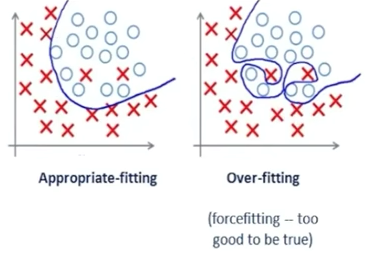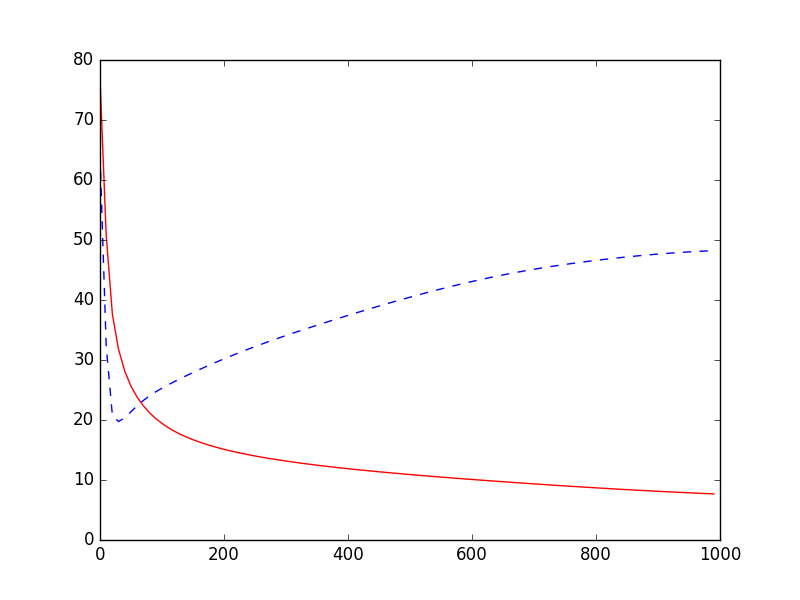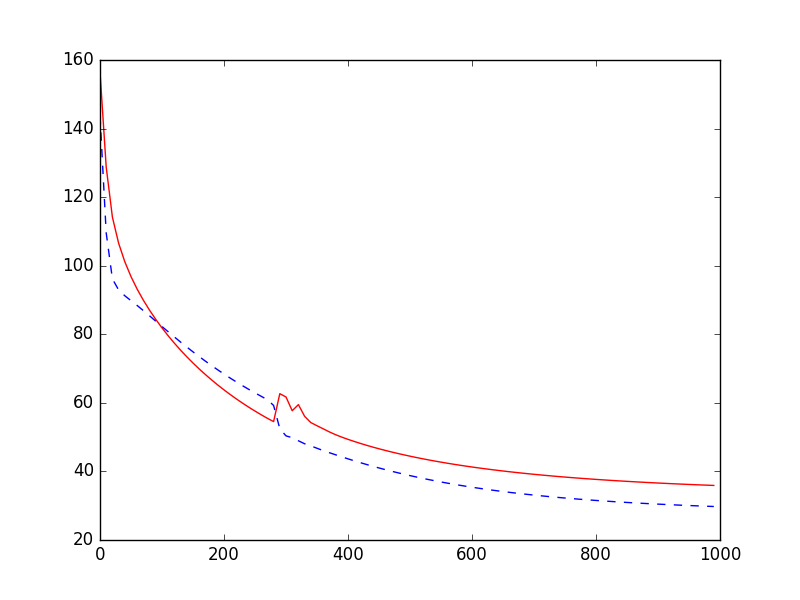# Regularization 正规化

## 要点 ¶## 导入模块并创建数据 ¶

import theano
from sklearn.datasets import load_boston
import theano.tensor as T
import numpy as np
import matplotlib.pyplot as plt


np.random.seed(100)
# minmax normalization, rescale the inputs
x_data = minmax_normalization(x_data)
y_data = load_boston().target[:, np.newaxis]


x 因为各个 feature 的取值范围区别较大，所以用 minmax_normalization 对数据进行归一化，这样可以把每个 feature 都压缩到 0-1 的范围。

def minmax_normalization(data):
xs_max = np.max(data, axis=0)
xs_min = np.min(data, axis=0)
xs = (1 - 0) * (data - xs_min) / (xs_max - xs_min) + 0
return xs


# cross validation, train test data split
x_train, y_train = x_data[:400], y_data[:400]
x_test, y_test = x_data[400:], y_data[400:]

x = T.dmatrix("x")
y = T.dmatrix("y")


## 建立模型 ¶

l1 = Layer(x, 13, 50, T.tanh)
l2 = Layer(l1.outputs, 50, 1, None)


# the way to compute cost
cost = T.mean(T.square(l2.outputs - y))      # without regularization
# cost = T.mean(T.square(l2.outputs - y)) + 0.1 * ((l1.W ** 2).sum() + (l2.W ** 2).sum())  # with l2 regularization
# cost = T.mean(T.square(l2.outputs - y)) + 0.1 * (abs(l1.W).sum() + abs(l2.W).sum())  # with l1 regularization

gW1, gb1, gW2, gb2 = T.grad(cost, [l1.W, l1.b, l2.W, l2.b])


## 激活模型 ¶

learning_rate = 0.01
train = theano.function(
inputs=[x, y],
updates=[(l1.W, l1.W - learning_rate * gW1),
(l1.b, l1.b - learning_rate * gb1),
(l2.W, l2.W - learning_rate * gW2),
(l2.b, l2.b - learning_rate * gb2)])

compute_cost = theano.function(inputs=[x, y], outputs=cost)


## 训练模型 ¶

train_err_list 等来记录训练过程中产生的误差，方便后面可视化。 训练 1000 次，每 10 步记录一次训练集和测试集的误差。

# record cost
train_err_list = []
test_err_list = []
learning_time = []

for i in range(1000):
train(x_train, y_train)
if i % 10 == 0:
# record cost
train_err_list.append(compute_cost(x_train, y_train))
test_err_list.append(compute_cost(x_test, y_test))
learning_time.append(i)


## 可视化结果 ¶

# plot cost history
plt.plot(learning_time, train_err_list, 'r-')
plt.plot(learning_time, test_err_list, 'b--')
plt.show()L2 正则化时，比上一个好很多，测试集的误差也在不断减小。